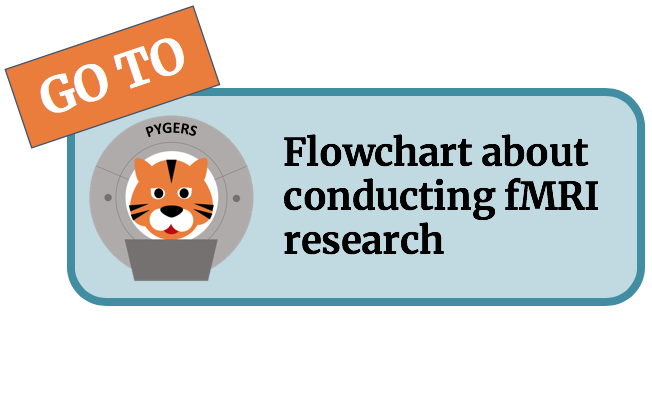# Input/output and file formats¶

```def load_epi_data(sub, ses, task):
return epi_data

```

If you want to open the actual image then add get_data() or get_fdata(), e.g.:

```mask.get_data()
```

```nifti_masker = NiftiMasker(mask_img=mask)
```nifti_masker = NiftiMasker(mask_img=mask,  high_pass=1/128, t_r=1.5)